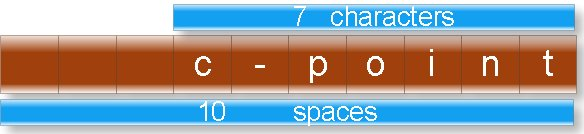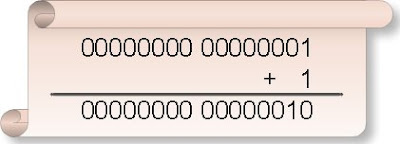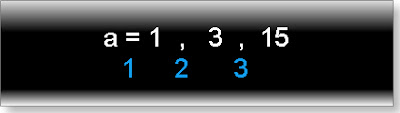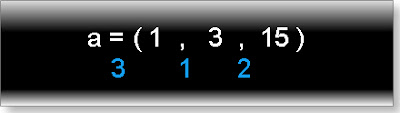## INDEX

### Tricky c questions and answers

Tricky c programs question for interview and answers with explanation. These questions are for experienced persons.

C advanced interview questions and answers

(1) What will be output if you will compile and execute the following c code?

struct marks{
int p:3;
int c:3;
int m:2;
};
void main(){
struct marks s={2,-6,5};
printf("%d %d %d",s.p,s.c,s.m);
}

(a) 2 -6 5
(b) 2 -6 1
(c) 2 2 1
(d) Compiler error
(e) None of these

Answer: (c)
Explanation:
Binary value of 2: 00000010 (Select three two bit)
Binary value of 6: 00000110
Binary value of -6: 11111001+1=11111010
(Select last three bit)
Binary value of 5: 00000101 (Select last two bit)

Complete memory representation:(2) What will be output if you will compile and execute the following c code?

void main(){
int huge*p=(int huge*)0XC0563331;
int huge*q=(int huge*)0xC2551341;
*p=200;
printf("%d",*q);
}

(a)0
(b)Garbage value
(c)null
(d) 200
(e)Compiler error

Answer: (d)
Explanation:
Physical address of huge pointer p
Huge address: 0XC0563331
Offset address: 0x3331
Segment address: 0XC056
Physical address= Segment address * 0X10 + Offset address
=0XC056 * 0X10 +0X3331
=0XC0560 + 0X3331
=0XC3891
Physical address of huge pointer q
Huge address: 0XC2551341
Offset address: 0x1341
Segment address: 0XC255
Physical address= Segment address * 0X10 + Offset address
=0XC255 * 0X10 +0X1341
=0XC2550 + 0X1341
=0XC3891
Since both huge pointers p and q are pointing same physical address so content of q will also same as content of q.

(3) Write c program which display mouse pointer and position of pointer.(In x coordinate, y coordinate)?

Answer:
#include”dos.h”
#include”stdio.h”
void main()
{
union REGS i,o;
int x,y,k;
//show mouse pointer
i.x.ax=1;
int86(0x33,&i,&o);
while(!kbhit()) //its value will false when we hit key in the key board
{
i.x.ax=3; //get mouse position
x=o.x.cx;
y=o.x.dx;
clrscr();
printf("(%d , %d)",x,y);
delay(250);
int86(0x33,&i,&o);
}
getch();
}

(4) Write a c program to create dos command: dir.

Answer:

Step 1: Write following code.

#include “stdio.h”
#include “dos.h”
void main(int count,char *argv[]){
struct find_t q ;
int a;
if(count==1)
argv="*.*";
a = _dos_findfirst(argv,1,&q);
if(a==0){
while (!a){
printf(" %s\n", q.name);
a = _dos_findnext(&q);
}
}
else{
printf("File not found");
}
}

Step 2: Save the as list.c (You can give any name)
Step 3: Compile and execute the file.
Step 4: Write click on My computer of Window XP operating system and select properties.
Step 5: Select Advanced -> Environment Variables
Step 6: You will find following window:
Click on new button (Button inside the red box)Step 7: Write following:
Variable name: path
Variable value: c:\tc\bin\list.c (Path where you have saved)Step 8: Open command prompt and write list and press enter.
Command line argument tutorial.

(6) What will be output if you will compile and execute the following c code?

void main(){
int i=10;
static int x=i;
if(x==i)
printf("Equal");
else if(x>i)
printf("Greater than");
else
printf("Less than");
}

(a) Equal
(b) Greater than
(c) Less than
(d) Compiler error
(e) None of above

Answer: (d)
Explanation:
static variables are load time entity while auto variables are run time entity. We can not initialize any load time variable by the run time variable.
In this example i is run time variable while x is load time variable.

(7) What will be output if you will compile and execute the following c code?

void main(){
int i;
float a=5.2;
char *ptr;
ptr=(char *)&a;
for(i=0;i<=3;i++)
printf("%d ",*ptr++);
}

(a)0 0 0 0
(b)Garbage Garbage Garbage Garbage
(c)102 56 -80 32
(d)102 102 -90 64
(e)Compiler error

Answer: (d)
Explanation:
In c float data type is four byte data type while char pointer ptr can point one byte of memory at a time.
Memory representation of float a=5.2ptr pointer will point first fourth byte then third byte then second byte then first byte.
Content of fourth byte:
Binary value=01100110
Decimal value= 64+32+4+2=102
Content of third byte:
Binary value=01100110
Decimal value=64+32+4+2=102
Content of second byte:
Binary value=10100110
Decimal value=-128+32+4+2=-90
Content of first byte:
Binary value=01000000
Decimal value=64
Note: Character pointer treats MSB bit of each byte i.e. left most bit of above figure as sign bit.

(8) What will be output if you will compile and execute the following c code?

void main(){
int i;
double a=5.2;
char *ptr;
ptr=(char *)&a;
for(i=0;i<=7;i++)
printf("%d ",*ptr++);
}

(a) -51 -52 -52 -52 -52 -52 20 64
(b) 51 52 52 52 52 52 20 64
(c) Eight garbage values.
(d) Compiler error
(e) None of these

Answer: (a)
Explanation:
In c double data type is eight byte data type while char pointer ptr can point one byte of memory at a time.
Memory representation of double a=5.2ptr pointer will point first eighth byte then seventh byte then sixth byte then fifth byte then fourth byte then third byte then second byte then first byte as shown in above figure.
Content of eighth byte:
Binary value=11001101
Decimal value= -128+64+8+4+1=-51
Content of seventh byte:
Binary value=11001100
Decimal value= -128+64+8+4=-52
Content of sixth byte:
Binary value=11001100
Decimal value= -128+64+8+4=-52
Content of fifth byte:
Binary value=11001100
Decimal value= -128+64+8+4=-52
Content of fourth byte:
Binary value=11001100
Decimal value= -128+64+8+4=-52
Content of third byte:
Binary value=11001100
Decimal value= -128+64+8+4=-52
Content of second byte:
Binary value=000010100
Decimal value=16+4=20
Content of first byte:
Binary value=01000000
Decimal value=64
Note: Character pointer treats MSB bit of each byte i.e. left most bit of above figure as sign bit.

(9) What will be output if you will compile and execute the following c code?

void main(){
printf("%s","c" "question" "bank");
}

(a) c question bank
(b) c
(c) bank
(d) cquestionbank
(e) Compiler error

Answer: (d)
Explanation:
In c string constant “xy” is same as “x” “y”

(10) What will be output if you will compile and execute the following c code?

void main(){
printf("%s",__DATE__);
}

(a) Current system date
(b) Current system date with time
(c) null
(d) Compiler error
(e) None of these

Answer: (a)
Explanation:
__DATE__ is global identifier which returns current system date.

(11) What will be output if you will compile and execute the following c code?

void main(){
char *str="c-pointer";
printf("%*.*s",10,7,str);
}

(a) c-pointer
(b) c-pointer
(c) c-point
(d) cpointer null null
(e) c-point

Answer: (e)
Explanation:
Meaning of %*.*s in the printf function:
First * indicates the width i.e. how many spaces will take to print the string and second * indicates how many characters will print of any string.
Following figure illustrates output of above code:(12) What will be output if you will compile and execute the following c code?

void start();
void end();
#pragma startup start
#pragma exit end
int static i;
void main(){
printf("\nmain function: %d",++i);
}
void start(){
clrscr();
printf("\nstart function: %d",++i);
}
void end(){
printf("\nend function: %d",++i);
getch();
}

(a)
main function: 2
start function: 1
end function:3
(b)
start function: 1
main function: 2
end function:3
(c)
main function: 2
end function:3
start function: 1
(d) Compiler error
(e) None of these

Answer: (b)
Explanation:
Every c program start with main function and terminate with null statement. But #pragma startup can call function just before main function and #pragma exit
(13) What will be output if you will compile and execute the following c code?

void main(){
int a=-12;
a=a>>3;
printf("%d",a);
}

(a) -4
(b) -3
(c) -2
(d) -96
(e) Compiler error

Answer :( c)
Explanation:
Binary value of 12 is: 00000000 00001100
Binary value of -12 wills 2’s complement of 12 i.e.So binary value of -12 is: 11111111 11110100Right shifting rule:
Rule 1: If number is positive the fill vacant spaces in the left side by 0.
Rule 2: If number is negative the fill vacant spaces in the left side by 1.
In this case number is negative. So right shift all the binary digits by three space and fill vacant space by 1 as shown following figure:Since it is negative number so output will also a negative number but its 2’s complement.Hence final out put will be:And its decimal value is: 2
Hence output will be:-2

(14) What will be output if you will compile and execute the following c code?

#include "string.h"
void main(){
clrscr();
printf("%d%d",sizeof("string"),strlen("string"));
getch();
}
(a) 6 6
(b) 7 7
(c) 6 7
(d) 7 6
(e) None of these

Answer: (d)
Explanation:
Sizeof operator returns the size of string including null character while strlen function returns length of a string excluding null character.

(15) What will be output if you will compile and execute the following c code?

void main(){
static main;
int x;
x=call(main);
clrscr();
printf("%d ",x);
getch();
}
int call(int address){
address++;
return address;
}
(a) 0
(b) 1
(c) Garbage value
(d) Compiler error
(e) None of these

Answer: (b)
Explanation:
As we know main is not keyword of c but is special type of function. Word main can be name variable in the main and other functions.
(16) What will be output if you will compile and execute the following c code?

void main(){
int a,b;
a=1,3,15;
b=(2,4,6);
clrscr();
printf("%d ",a+b);
getch();
}
(a) 3
(b) 21
(c) 17
(d) 7
(e) Compiler error

Answer: (d)
Explanation:
In c comma behaves as separator as well as operator.
a=1, 3, 15;
b= (2, 4, 6);
In the above two statements comma is working as operator. Comma enjoys least precedence and associative is left to right.
Assigning the priority of each operator in the first statement:Hence 1 will assign to a.
Assigning the priority of each operator in the second statement:(17) What will be output if you will compile and execute the following c code?

int extern x;
void main()
printf("%d",x);
x=2;
getch();
}
int x=23;

(a) 0
(b) 2
(c) 23
(d) Compiler error
(e) None of these

Answer: (c)
Explanation:
extern variables can search the declaration of variable any where in the program.

(18) What will be output if you will compile and execute the following c code?

void main(){
int i=0;
if(i==0){
i=((5,(i=3)),i=1);
printf("%d",i);
}
else
printf("equal");
}

(a) 5
(b) 3
(c) 1
(d) equal
(e) None of above

Answer: (c)
Explanation:

(19) What will be output if you will compile and execute the following c code?

void main(){
int a=25;
clrscr();
printf("%o %x",a,a);
getch();
}

(a) 25 25
(b) 025 0x25
(c) 12 42
(d) 31 19
(e) None of these

Answer: (d)
Explanation:
%o is used to print the number in octal number format.
%x is used to print the number in hexadecimal number format.
Note: In c octal number starts with 0 and hexadecimal number starts with 0x.

(20) What will be output if you will compile and execute the following c code?

#define message "union is\
power of c"
void main(){
clrscr();
printf("%s",message);
getch();
}

(a) union is power of c
(b) union ispower of c
(c) union is
Power of c
(d) Compiler error
(e) None of these

Answer: (b)
Explanation:
If you want to write macro constant in new line the end with the character \.

(21) What will be output if you will compile and execute the following c code?

#define call(x) #x
void main(){
printf("%s",call(c/c++));
}

(a)c
(b)c++
(c)#c/c++
(d)c/c++
(e)Compiler error

Answer: (d)
Explanation:
# is string operator. It converts the macro function call argument in the string. First see the intermediate file:
test.c 1:
test.c 2: void main(){
test.c 3: printf("%s","c/c++");
test.c 4: }
test.c 5:
It is clear macro call is replaced by its argument in the string format.

(22) What will be output if you will compile and execute the following c code?

void main(){
if(printf("cquestionbank"))
printf("I know c");
else
printf("I know c++");
}

(a) I know c
(b) I know c++
(c) cquestionbankI know c
(d) cquestionbankI know c++
(e) Compiler error

Answer: (c)
Explanation:
Return type of printf function is integer which returns number of character it prints including blank spaces. So printf function inside if condition will return 13. In if condition any non- zero number means true so else part will not execute.

If you have any doubt in above Tricky questions with explanation you can ask through comment section.

#### 63 comments:Anonymous said...

nice questions and answers

ganesh said...

good qustionsAnonymous said...

good question as well ans
but i didnt got explanation of qustion 16
so please elborate explanation if possibe

Ballu Bisht said...

this is really a vry good stuff..Anonymous said...

good questions :) thanksAnonymous said...

thanks :) good questions

Piyush Shrivastava said...

Nice collection here. I am an engineering student and these questions will sure help in building up my programming aptitude. Thanks

amit said...

can someone please elaborate about question no.16

sandeep yadav said...

very very good questions...

adarsh said...

ON question 16:
when i=2,3,4;//i.e without brackets
priority in given to first one.

when i=(2,3,4)//i.e with brackets
priority is given to last one

amit said...

I'm very much greatful to you adarsh for your clarification.......

amit said...

I'm very greatful to you for your clarification.Anonymous said...

(6) What will be output if you will compile and execute the following c code?

void main(){
int i=10;
static int x=i;
if(x==i)
printf("Equal");
else if(x>i)
printf("Greater than");
else
printf("Less than");
}

This code perefctly compiles in C++ giving answer "EQUAL"Anonymous said...

Thanks for Qs :) really a good set of Qs for experienced people.
Can anyone share some more interesting Qs like this.Anonymous said...

explaination for 16th ques?

deepak chandra pandey(rit intitute) said...

first we use clrscr()functionafter main function......in 16...the value of a and b in ram....then we useing clrscr....which used clear the memory.....printf not found any value and give it compile time error...........

deepak chandra pandey(rit intitute) said...

brackets means starting of stack.....means last in first out(LIFO)

Yadhunandana R K said...

challenging questions that floating storage question is awesome.........

rajendra kumar padhy said...

nice questions..............
this will help me a lot.

Dineshvenkat S said...

Will you please explain the 3rd program mouse pointer

madhu said...

1,2,3,4,5,1,2,3,1,2,3,4,5......
separate the series like
1,,2,3,4,5 and a,2,3
and 1,2,3,4,5
ten find the sum of this series .. guys please help me ......

madhu said...

write a program to find the power of 12 without using pow function ?

the output should be in the form of

2 power 12

3 power 12...so on ...Anonymous said...

int powi(int number, int exponent)
{
int i, product = 1;
for (i = 0; i < exponent; i++)
product *= number;

return product;
}

void main()
{
int rez=0,i=0;
for(i=0;i<5;i++)
{
rez=powi(12,i);
printf("\n%d power of 12 is %d",i,rez);
}

}Dr.Sexpert said...

Not in getting laid. {()}---<--8Grammar Nazi said...

*explanation

shanu aakash said...

why array index starts with zero

pankaj verma said...

why this code gives output :
-2 -1
instead of
2 1

#include
struct marks {
int a:1;
int b:2;
};

int main(){
struct marks obj={1,6};
printf("%d %d\n",obj.b,obj.a);

}

pankaj verma said...

#include
long int power(int ,int );

int main(){
int i,n;
printf("enter a number(not greater than 5 other wise overflow will occer.) to generate power series :\n");
scanf("%d",&n);
for(i=1;i<=n;i++)
printf("%d power 12 is : %ld\n",i,power(i,12));
}

long int power(int a,int b){
int c;
long int ans=1;
for(c=1;c<=b;c++)
ans=ans*a;
return ans;
}Anonymous said...

In "C" it will give compilation error, whereas in C++ it will give "EQUAl"

P@m said...

thanks itz really helpfull :)Anonymous said...

void start();
void end();
#pragma startup start
#pragma exit end
int static i;
void main(){
printf("\nmain function: %d",++i);
}
void start(){
clrscr();
printf("\nstart function: %d",++i);
}
void end(){
printf("\nend function: %d",++i);
getch();
}

On Fedora16 it will print output as:

main function:1

Sathish said...

Very good stuff... Thanks :-0

neerajkumarghildiyal said...

really very intresting sirrrrrrrrrrrrrr.........Anonymous said...

@pankaj verma
for b there are only 2 bits allocated so 110 ->10 first bit(most sig) 1 so -ve =>-2
and for a there is only 1 bit alloc so 1->1 first bit(most sig) 1 so -ve hence it => -1

Pramit Ghosh said...

1 in your program can be represented in binary by 00000001..And 6 is as 00000110..Now using a:1 means taking the single lsb i.e 1 which also represents negative sign..alternativly if you use a:2,your obj.a will be +1..Again,for 6,b:2 i,e taking the 2 lsb's from 6 which is 10 i.e representation of -2,alternatively using b:4 would mean taking 4 lsb's as 0110,so obj.b woild be 6..

Vikram Singh said...

very good stuff

vikas magar said...

The following C program segfaults of IA-64, but works fine on IA-32.

int main()
{
int* p;
p = (int*)malloc(sizeof(int));
*p = 10;
return 0;
}

Why does it happen so?

vikas magar said...

What is the differnce between the following function calls to
scanf?(Please notice the space carefully in the second call. Try
removing it and observe the behaviour of the program)
#include
int main()
{
char c;
scanf("%c",&c);
printf("%c\n",c);

scanf(" %c",&c);
printf("%c\n",c);

return 0;
}

vikas magar said...

What is the use of the following macro?
#define DPRINTF(x) printf("%s:%d\n",#x,x)

ece said...

could i get answer for the following question.

Problem;
Given an English text print out the list of words and their anagrams in their order of occurrence. Word A is an anagram of word B if it can be derived from word B by merely shuffling the letters in word B.

Important Points:

Text can contain words in upper case as well as lower case and punctuation marks

Anagrams are not to be case sensitive

The word and its anagrams are to be printed in the order of their occurrence in the text, separated by a blank.

If a word has no anagram in the text, then do not print it

If a word or its anagram occurs more than once do not print it again

Numbers are to be considered as valid words

Input:

A text containing K English words (where K <= 5000), with spaces and punctuation marks

Output:

The output should contain the word (in its order of occurrence in the text) and its set of anagrams in the text (again in their order of occurrence), separated by blanks

Each new list of words and anagrams should begin on a new line

All words in the output should be printed in lower case characters

Sample Input

Sample Output

Parts of the world have sunlight for close to 24 hours during summer. Dan went to the north pole to lead an expedition during summer. He had a strap on his head to identify himself as the leader. Dan had to deal with the sun never going down for 42 consecutive days and his leadership strap soon became a blindfold. He wondered what kind of traps lay ahead of him.

parts strap traps

24 42

dan and

lead deal

Mohit Kumar said...

Very good questions.........hope to see more in future!!!!Anonymous said...

int
rpow(int num, int power)
{
return power == 0 ? 1 : num * rpow(num, power-1);
}

main()
{
int num, power;
printf("Enter number : ");
scanf("%d", &num);
printf("to +ve power : ");
scanf("%d", &power);
if (power < 0) {
printf("power must be +ve");
exit(-1);
}
printf("number %d raised to power %d is %d\n", num, power, rpow(num, power));
}

neerajkumarghildiyal said...

sir plz.. add tutorials for file handling in c also.Anonymous said...

good work

dishank pant said...

explain the output of following question??
#include
int main()
{
int a=-10;
char *p=(char *)&a;
printf("%d",*p);
return 0;
}

rohit sharma said...

Doubt in
void main(){
int i;
double a=5.2;
char *ptr;
ptr=(char *)&a;
for(i=0;i<=7;i++)
printf("%d ",*ptr++);
}Anonymous said...

yeasuuuuuuuvijay kumar said...

thanks for logical informationAnonymous said...

Really Helpful questions...good coverage of all topics...Thanks a lot!Anonymous said...

kusuuuuuuuuuuuuuuuuuuuuuuu

Himanshu said...

use fflush(); just before the 2nd scanf function...

Shelvane Baburao said...

int main()
{
int a=15;
a=(++a)+015+0x15;
printf("%d",a);
return 1;
}

i don't know how to work octal and hex hear so plz tell me what is o/p with explanation of that prog.

Shravan kashyap said...

Hello!, could you plz explain me the precedence order in 16th question , especially II case i..e a=(1,2,3),
in a much more clear way.
Thank you

thulasi said...

This site will gives more time pass for lovers of C, and can love C, in deep.

Nagaraju muthyala said...

ITS GOOD FOR US

Rahul Barahpuria said...

binary form of int value 10 is 00000000 00001010 (2Bytes)
binary form of -10 is its 2's compliment i.e., 11111111 11110110

Now, size of char data type is 1 byte only,
so, *p will have value 11110110 only. (11111111 is excluded)
Negative form of 11110110 is its 2's compliment i,e. 00001010 whose decimal value is -10 which will be shown as the output.

Archana NA said...

i = a, b, c;// stores a into i, discarding the unused b and c rvalues
i = (a, b, c);// stores c into i, discarding the unused a and b rvalues

Comma works as an operator in first case. Precedence of comma operator is least in operator precedence table. So the assignment operator takes precedence over comma and the expression “i = a, b, c″ becomes equivalent to “(i = a), b, c″.

In the second case,brackets are used.So comma operator is executed first.(a, b, c) is a sequence of expressions, separated by commas, which evaluates to the last expression c.

Hope this helps!

Archana NA said...

i = a, b, c;// stores a into i, discarding the unused b and c rvalues
i = (a, b, c);// stores c into i, discarding the unused a and b rvalues

Comma works as an operator in first case. Precedence of comma operator is least in operator precedence table. So the assignment operator takes precedence over comma and the expression “i = a, b, c″ becomes equivalent to “(i = a), b, c″.

In the second case,brackets are used.So comma operator is executed first.(a, b, c) is a sequence of expressions, separated by commas, which evaluates to the last expression c.

Hope this helps!

Archana NA said...

50
The statement mixes decimal,octal(o)and hexadecimal(ox).
Before addition,everything will be converted to binary format.

aditya patel said...

very helpful site i love this

surya kalvas said...

Suggest me a book for these type of tricky problems of c

kelin chen said...

This website can live streaming , you can join at my site :
agen judi online terpercaya
Prediksi Bola

Thank you
agenpialaeropa.club
gamesonline.ga
beritasemasaterikini.netAnonymous said...

Find some more C Tricky Questions on interviewquestions.guru